407 493 6601

# High School Geometry - Similar Polygons

## Introduction

• The prerequisite for any two polygons to be considered for the test of similarity is that they must be of the same shape (i.e. both the polygons must have the same number of sides).
• For example, a triangle cannot be compared with a quadrilateral for the test of similarity as they have different shapes.
• Two polygons of the same number of sides are similar if and only if:

(i) their corresponding angles are equal, and

(ii) their corresponding sides are proportion (in the same ratio)

• For any two polygons to be similar, the above-mentioned conditions must be satisfied.
• Merely satisfying only one condition does not guarantee the polygons to be similar. The figures shown below demonstrate the same fact.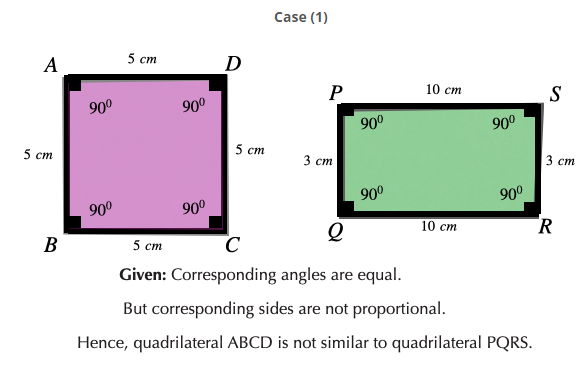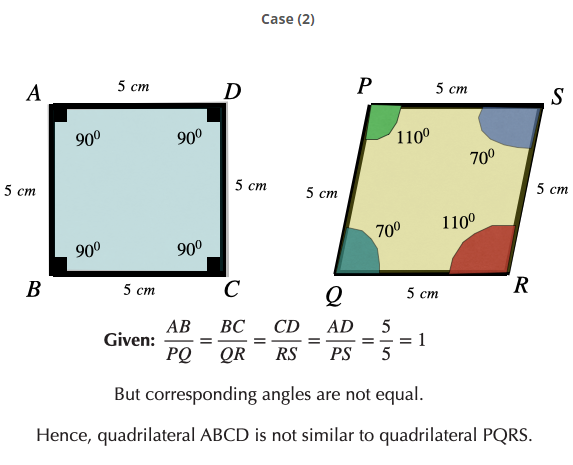• Two triangles are said to be similar if
1. their corresponding angles are equal and
2. their corresponding sides are proportional [i.e. the ratio of the length of their corresponding sides are same]
• Thus, we can say that similar triangles have the same shape but may not be equal in size.
• The similarity of triangles is denoted by the symbol '$~$'. It is different from the symbol of congruence i.e. '$\cong$'.
• Consider the two triangles as shown in the figure below.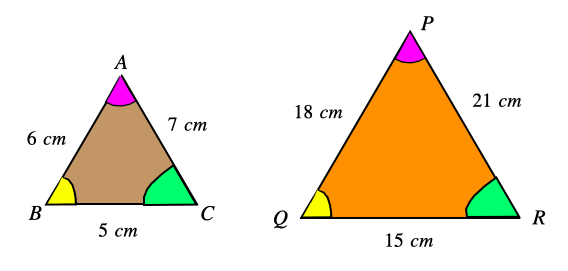Here, $\angle A=\angle P$$\angle B=\angle Q$ and $\angle C=\angle R$. Also $\frac{AB}{PQ}=\frac{BC}{QR}=\frac{AC}{PR}=3$. Hence $△ABC~△PQR$.

## Criteria for Triangle Similarity and Some Theorems on Similar Polygons

Two triangles can be proved similar to each other by some criteria, which are as follows:

• Angle-Angle-Angle (AAA) Similarity
• Side-Side-Side (SSS) Similarity
• Side-Angle-Side (SAS) Similarity

1) Angle-Angle-Angle (AAA) Similarity:

If in two triangles, corresponding angles are equal, then their corresponding sides are in the same ratio (or proportion), and hence the two triangles are similar.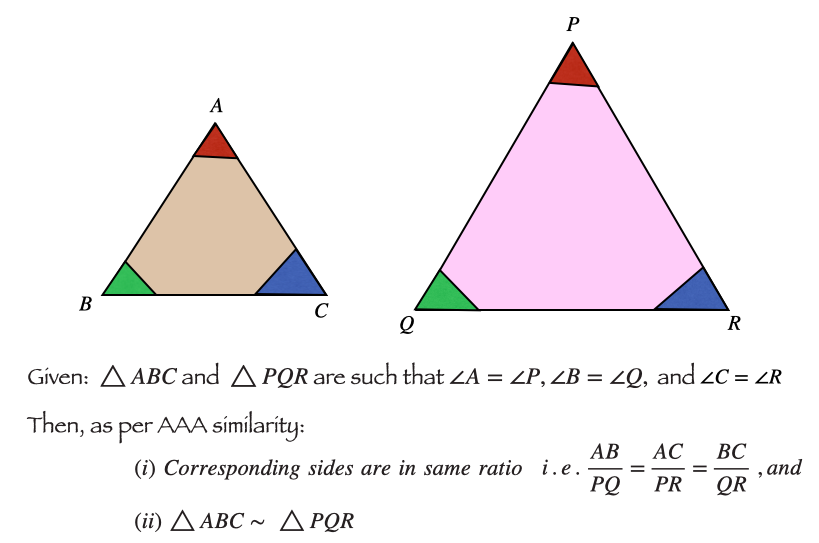2) Side-Side-Side (SSS) Similarity

If, in two triangles, the sides of one triangle are proportional to (i.e. in the same ratio of ) the sides of the other triangle, then their corresponding angles are equal, and hence the two triangles are similar.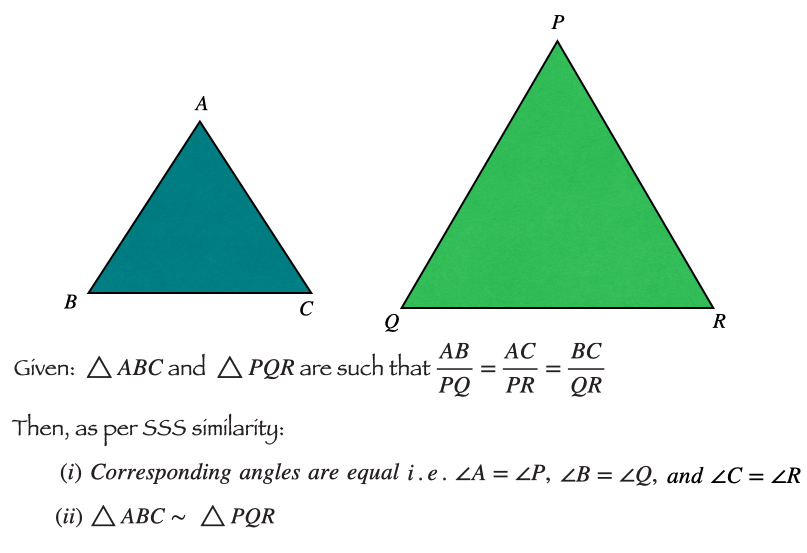3) Side-Angle-Side (SAS) Similarity

If one angle of a triangle is equal to one angle of the other triangle and the sides including these angles are proportional, then the two triangles are similar.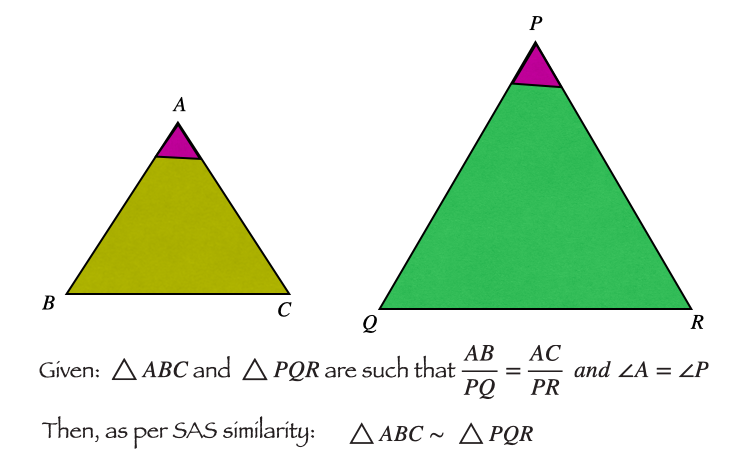Other Theorems Related to Similarity.

1. Proportional Segments Theorem (or Triangle Proportionality Theorem): If a line intersects the two sides of a triangle at distinct points and is parallel to the third side, then the line divides the two sides into two proportional segments. Refer to the figure below: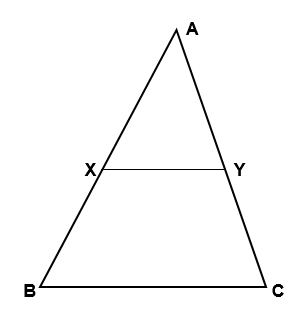If $XY\parallel BC$, then and $\frac{AY}{AC}=\frac{XY}{BC}$.

If $XY\parallel BC$, then $△AXY~△ABC.$

2. Converse of the Proportional Segments Theorem: If a line intersects the two sides of a triangle at distinct points and cuts these sides into proportional segments, then the line is parallel to the third side.

3. The bisector of an angle of a triangle divides the opposite side into segments that are proportional to the adjacent sides.

Refer to the figure below: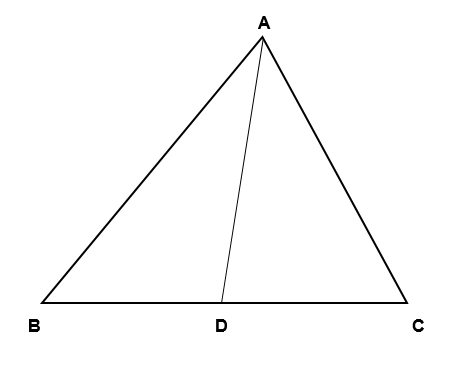In  is an angle bisector of $\angle BAC$

$\frac{AB}{AC}=\frac{BD}{CD}$

4. Corresponding medians of similar triangles are proportional to the corresponding sides. Refer to the figure below: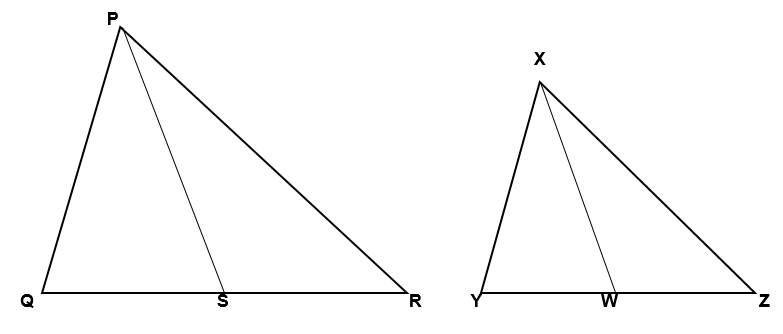$△PQR~△XYZ$is a median to $QR$ and is a median to $YZ$.

and $\frac{PS}{XW}=\frac{QR}{YZ}$

5. Corresponding altitudes of similar triangles are proportional to the corresponding sides. Refer to the figure below: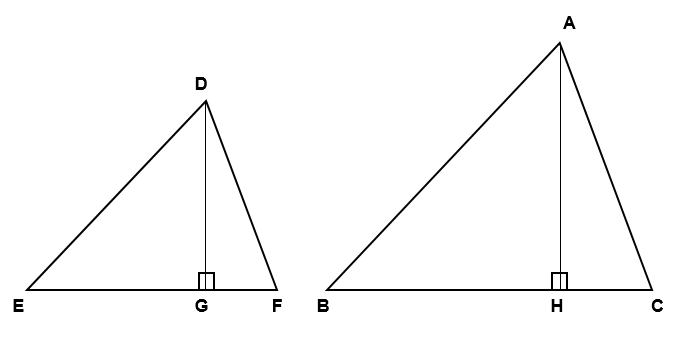$△DEF~△ABC$ with $DG$ and $AH$ are altitudes.

and $\frac{DG}{AH}=\frac{EF}{BC}$.

6. The ratio of the perimeters of two similar triangles or two similar polygons is the same as the ratio of any two corresponding sides.

7. If two triangles or two polygons are similar, then the ratio of their areas is equal to the square of the ratio of any two corresponding sides.

8. If two triangles are similar, then the ratio of their areas is equal to the square of the ratio of their perimeters.

## Solved Examples

Example 1: In the given figure, pentagon $ABCDE$ is similar to pentagon $PQRST$. If the corresponding angles are equal and the length of sides and , then find the value of $x$.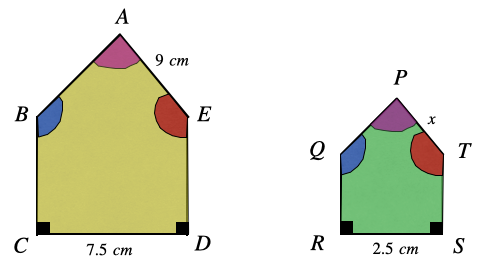Solution: We know if two polygons are similar, the ratios of each pair of their corresponding sides are equal.

So, $\frac{CD}{RS}=\frac{AE}{PT}$

or, $\frac{7.5}{2.5}=\frac{9}{x}⇒x=\frac{9×2.5}{7.5}=3$

Therefore, the value of $x$ is cm.

Example 2: Check whether the given pair of triangles shown in the figure are similar. Also, specify the similarity criteria (if they are similar).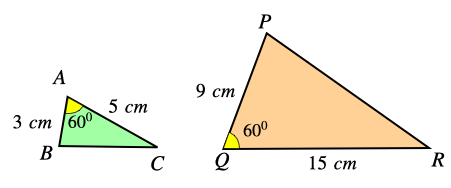Solution: Here $\frac{AB}{QP}=\frac{3}{9}=\frac{1}{3}$ and $\frac{AC}{QR}=\frac{5}{15}=\frac{1}{3}$. Also, the included angle is equal i.e. $\angle A=\angle Q$.

As per the SAS similarity criterion, if one angle of a triangle is equal to one angle of the other triangle and the sides including these angles are proportional, then the two triangles are similar.

Hence, we can say that $△ABC~△QPR$ by SAS similarity.

Example 3: Triangles RAM and PET are similar triangles, as shown in the figure below. Find the length of PT.